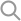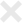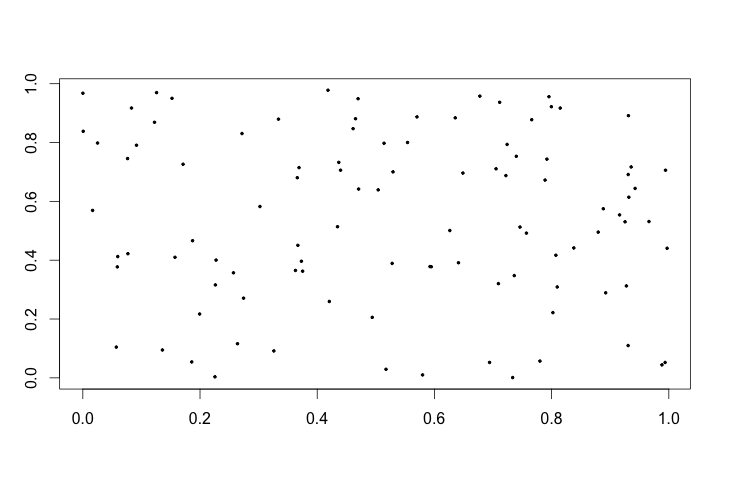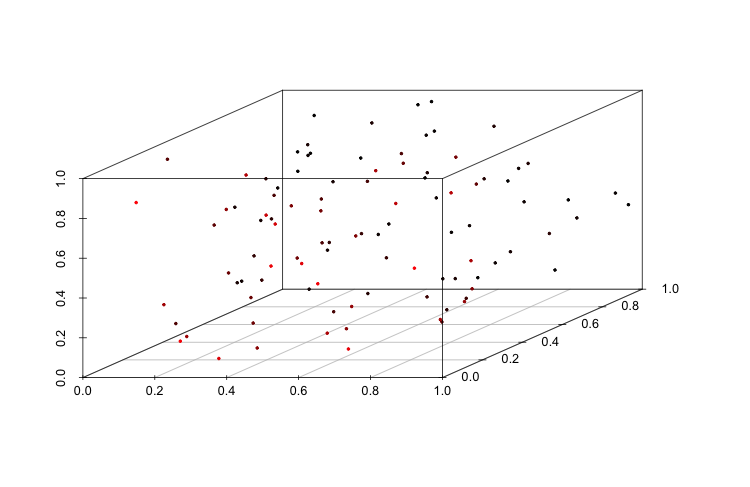Project Predictionio has retired. For details please refer to its Attic page.The purpose of this guide is to teach developers how to incorporate "dimensionality reduction" into a PredictionIO engine Principal Component Analysis (PCA) on the MNIST digit recognition dataset. To do this, you will be modifying the PredictionIO classification engine template. This guide will demonstrate how to import the specific data set in batch, and also how to change the engine components in order to incorporate the new sample data and implement PCA.

In machine learning, specifically in supervised learning, the general problem at hand is to predict a numeric outcome $$y$$ from a numeric vector $$\bf{x}$$. The different components of $$\bf{x}$$ are called features, and usually represent observed values such as a hospital patient's age, weight, height, sex, etc. There are subtle issues that begin to arise as the number of features contained in each feature vector increases. We briefly list some of the issues that arise as the number of features grows in size:

• Computation: The time complexity of machine learning algorithms often times depends on the number of features used. That is, the more features one uses for prediction, the more time it takes to train a model.

• Prediction Performance: Often times there will be features that, when used in training, will actually decrease the predictive performance of a particular algorithm.

• Curse of Dimensionality: It is harder to make inference and predictions in high dimensional spaces simply due to the fact that we need to sample a lot more observations. Think about it in this way, suppose that we sample 100 points lying on a flat solid square, and 100 points in a solid cube. The 100 points from the square will likely take up a larger proportion of its area, in comparison to the proportion of the cube's volume that the points sampled from it occupy. Hence we would need to sample more points from the cube in order to get better estimates of the different properties of the cube, such as height, length, and width. This is shown in the following figure:

100 Points Sampled From Unit Square 100 Points Sampled From Unit CubeDimensionality reduction is the process of applying a transformation to your feature vectors in order to produce a vector with the same or less number of features. Principal component Analysis (PCA) is a technique for dimensionality reduction. This can be treated as a data processing technique, and so with respect to the DASE framework, it will fall into the Data Preparator engine component.

This guide will also help to solidify the concept of taking an engine template and customizing it for a particular use case: hand-written numeric digit recognition.

## Data Example

As a guiding example, a base data set, the MNIST digit recognition dataset, is used. This is a perfect data set for dimensionality reduction, for, in this data set, the features that will be used for learning are pixel entries in a $$28 \times 28$$ pixel image. There is really no direct interpretation of any one feature, so that you do not lose anything in applying a transformation that will treat the features as linear combinations of some set "convenient" vectors.

Now, we first pull the classification engine template via the following bash line

 1 git clone https://github.com/apache/predictionio-template-attribute-based-classifier.git 

You should immediately be prompted with the following message:

 1 Please enter the template's Scala package name (e.g. com.mycompany): 

Go ahead and input FeatureReduction, and feel free to just press enter for the remaining message prompts. For the remainder of this guide, you will be working in your new engine directory, so go ahead and cd into your new engine directory. At this point, go ahead and run the command

 1 pio build 

This will make sure that the PredictionIO dependency version for your project matches the version installed on your computer. Now, download the MNIST train.csv data set from the link above, and put this file in the data directory contained in the new engine directory.

### Optional: Visualizing Observations

If you want to actually convert the observation pixel data to an image go ahead and create a Python script called picture_processing.py into your data directory and copy and paste the following code into the script:

 1 2 3 4 5 6 7 8 9 10 11 12 13 14 15 16 17 18 19 20 21 22 from PIL import Image import sys obs_num = int(sys.argv) f = open('./data/train.csv', 'r').read().split('\n') var_names = f.split(',') f = f[1 : -1] f = [list(map(int, x[1 : ])) for x in (y.split(",") for y in f)] def create_image(pixel_array): img = Image.new('RGB', (28, 28)) pixels = img.load() count = 0 for i in range(img.size): for j in range(img.size): pixels[i, j] = (i, j, pixel_array[count]) count += 1 return img create_image(f[obs_num]).show() 

To use this run the following line:

 1 python data/picture_processing.py k 

where you will replace k with an integer between 0 and 41999 (referring to an observation number). This script uses the Python pillow library, and, if you have it installed, the above command should open up a window with an image of a hand-written numerical digit.

### Importing the Data

You will use the PredictionIO Python SDK to prepare the data for batch import. Go ahead and create a Python script called export_events.py in the same data directory, and copy and paste the following code:

 1 2 3 4 5 6 7 8 9 10 11 12 13 14 15 16 17 18 19 20 21 22 23 24 25 26 27 28 29 30 31 32 33 34 35 """ Import digit recognition data. """ import predictionio import argparse import pytz from datetime import datetime ### Remove the variable name line, and last line. f = open("./data/train.csv", "r").read().split("\n")[1 : -1] ### Separate your observations into a tuple (label, pixel list). f = [(int(x), list(map(int, x[1 : ]))) for x in (y.split(",") for y in f)] ### JSON event exporter. exporter = predictionio.FileExporter("./data/digits.json") count = 0 print("Exporting events to JSON batch file........") for elem in f: exporter.create_event( event="digitData", entity_type="digit", entity_id=str(count), # use the count num as user ID properties= { "label":elem, "features":str(elem)[1 : -1] }, event_time = datetime.now(pytz.utc) ) count += 1 print("Exported {} events.".format(str(count))) 

This will import the data into the event server in a manner that will facilitate its processing in the Classification engine, although you will also need to modify the engine accordingly. In your new engine directory, run the above script via the following:

 1 python data/export_events.py 

This will create a file digits.json in your engine data directory. We will create a new application called FeatureReduction via the command:

 1 pio app new FeatureReduction 

This will create an application associated to an application ID and an access key. To import the data, you use the command in your engine directory:

 1 pio import --appid --input data/digits.json 

If the data has been successfully imported, you should see output of the form:

 1 2 3 4 5 ... [INFO] [Remoting] Starting remoting [INFO] [Remoting] Remoting started; listening on addresses :[akka.tcp://sparkDriver@10.0.0.30:65523] [INFO] [FileToEvents$] Events are imported. [INFO] [FileToEvents$] Done. 

The data is now in the event server.

## Principal Component Analysis

PCA begins with the data matrix $$\bf X$$ whose rows are feature vectors corresponding to a set of observations. In our case, each row represents the pixel information of the corresponding hand-written numeric digit image. The model then computes the covariance matrix estimated from the data matrix $$\bf X$$. The algorithm then takes the covariance matrix and computes the eigenvectors that correspond to its $$k$$ (some integer) largest eigenvalues. The data matrix is then mapped to the space generated by these $$k$$ vectors, which are called the $$k$$ principal components of $$\bf X$$. What this is doing is mapping the data observations into a lower-dimensional space that explains the largest variability in the data (contains the most information). The algorithm for implementing PCA is listed as follows:

### PCA Algorithm

Input: $$N \times p$$ data matrix $$\bf X$$; $$k \leq p$$, the number of desired features.

1. For each column in the data matrix: compute the average of all the entries contained in the column, and then subtract this average from each of the column entries.

2. Compute the $$k$$ eigenvectors corresponding to the $$k$$ largest eigenvalues of the matrix obtained in the first step.

Output: $$p \times k$$ matrix $$P$$ whose $$k$$ rows are the eigenvectors computed in the second step.

Now, to transform a $$p \times 1$$ feature vector $$\bf {x}$$, you multiply by the matrix $$P^T$$. Now, the vector $$P^T {\bf x}$$ is a feature vector with only $$k$$ components, which has accomplished the desired dimensionality reduction. Also, as a side note, the first step in the algorithm reduces the covariance matrix computation to that of only performing SVD on matrix obtained from step 1, which is numerically preferred, and necessary to extract the required eigenvectors.

## Modifying the Engine Template

We will be modifying the engine template by first re-defining our Query class located in the Engine.scala script as follows:

 1 2 3 class Query( val features : String ) extends Serializable 

We will continue to make the required engine modifications by following the DASE workflow. The next step is then to modify the engine's DataSource class which is the engine component in charge of reading the data from the event server.

### Data Source Modifications

The following changes will be made to the DataSource class. We will redefine the method readTraining as follows:

 1 2 3 4 5 6 7 8 9 10 11 12 13 14 15 16 17 18 ... override def readTraining(sc: SparkContext): TrainingData = { val data : RDD[Observation] = PEventStore.find( appName = dsp.appName, entityType = Some("digit"), eventNames = Some(List("digitData")) )(sc).map(e => Observation( e.properties.get[Double]("label"), e.properties.get[String]("features") )) new TrainingData(data) } ... 

This is essentially just making sure that the entityType, eventName, and properties fields match those specified in the script export_events.py. Also, a new class is introduced called Observation to serve as a wrapper for each data point's response and feature attributes, and the TrainingData is modified to hold an RDD of type Observation (instead of LabeledPoints):

 1 2 3 4 5 6 7 8 case class Observation ( label : Double, features : String ) class TrainingData( val observations: RDD[Observation] ) extends Serializable 

This also means that the readEval method must be redefined in a similar fashion:

 1 2 3 4 5 6 7 8 9 10 11 12 13 14 15 16 17 18 19 20 21 22 23 24 25 26 27 28 29 30 31 32 33 34 35 36 37  override def readEval(sc: SparkContext) : Seq[(TrainingData, EmptyEvaluationInfo, RDD[(Query, ActualResult)])] = { require(dsp.evalK.nonEmpty, "DataSourceParams.evalK must not be None") // The following code reads the data from data store. It is equivalent to // the readTraining method. We copy-and-paste the exact code here for // illustration purpose, a recommended approach is to factor out this logic // into a helper function and have both readTraining and readEval call the // helper. val data : RDD[Observation] = PEventStore.find( appName = dsp.appName, entityType = Some("digit"), eventNames = Some(List("digitData")) )(sc).map(e => Observation( e.properties.get[Double]("label"), e.properties.get[String]("features") )).cache // End of reading from data store // K-fold splitting val evalK = dsp.evalK.get val indexedPoints: RDD[(Observation, Long)] = data.zipWithIndex() (0 until evalK).map { idx => val trainingPoints = indexedPoints.filter(_._2 % evalK != idx).map(_._1) val testingPoints = indexedPoints.filter(_._2 % evalK == idx).map(_._1) ( new TrainingData(trainingPoints), new EmptyEvaluationInfo(), testingPoints.map { p => (new Query(p.features), new ActualResult(p.label)) } ) } } 

The motivation for defining the Observation class is to make it easy to maintain the format of the data as it was imported, and to help you look at each RDD element as a data observation in its original format. All of the data processing will be taken care of via the Preparator class.

### Preparator Modifications

Remember that the Data Preparator is the engine component that takes care of the necessary data processing prior to the fitting of a predictive model in the Algorithm component. Hence this stage is where you will implement PCA.

To make sure there is no confusion, replace the import statements in the Preparator.scala script with the following:

 1 2 3 4 5 6 7 import org.apache.predictionio.controller.{Params, PPreparator} import org.apache.spark.SparkContext import org.apache.spark.mllib.feature.{StandardScaler, StandardScalerModel} import org.apache.spark.mllib.linalg.distributed.RowMatrix import org.apache.spark.mllib.linalg.{DenseVector, Vectors, Vector} import org.apache.spark.rdd.RDD import org.apache.spark.mllib.regression.LabeledPoint 

Also, note that the PCA algorithm requires you to specify the hyperparameter $$k$$, or the desired number of features. Thus you will first define a parameter class PreparatorParams:

 1 2 3 case class PreparatorParams ( numFeatures : Int ) extends Params 

The next step is to implement the algorithm discussed in the above digression. This will all be done in the PreparedData class.

Remember that the classes Observation and Query store the pixel features as a string separated by ", ". Hence, for data processing, you first need a function, string2Vector, that will transform the feature strings to vectors. Now, you will need a function, scaler, that centers your observations (step 1 in PCA algorithm). Luckily, the StandardScaler and StandardScalerModel classes implemented in Spark MLLib can easily take care of this for you. The last part will be to actually compute the SVD of the data matrix which can also be easily done in MLLib. All this will be implemented in the PreparedData class which you will redefine as follows:

 1 2 3 4 5 6 7 8 9 10 11 12 13 14 15 16 17 18 19 20 21 22 23 24 25 26 27 28 29 30 31 32 33 34 35 36 class PreparedData( val data : RDD[Observation], val pp : PreparatorParams ) extends Serializable { /// Data Transformation Tools // Transform features string member to a MLLib Vector. private val string2Vector : (String => Vector) = (e : String) => Vectors.dense( e.split(", ").map(_.toDouble) ) // Create function for centering data. private val scaler : StandardScalerModel = new StandardScaler(true, false).fit( data.map(e => string2Vector(e.features)) ) // Compute PCA output matrix. private val pcaMatrix = new RowMatrix(data.map( e => string2Vector(e.features) )).computePrincipalComponents(pp.numFeatures).transpose /// Observation transformation. def transform (features : String): Vector = { pcaMatrix.multiply( new DenseVector(scaler.transform(string2Vector(features)).toArray) ) } // Data for inputting into learning Algorithm. val transformedData : RDD[LabeledPoint] = data.map(e => LabeledPoint( e.label, transform(e.features) )) } 

The function transform takes the string features and outputs a post-PCA feature vector. This is not made a private class member since it must also be used in transforming future queries. The member transformedData is the data set represented as an object that can be simply thrown into a classification model!

The final step is to incorporate the PreparatorParams into the Preparator class. This requires very little editing:

 1 2 3 4 5 6 class Preparator (pp: PreparatorParams) extends PPreparator[TrainingData, PreparedData] { def prepare(sc: SparkContext, trainingData: TrainingData): PreparedData = { new PreparedData(trainingData.observations, pp) } } 

The Data Preparator engine component is now complete, and we can move on to the Algorithm component.

### Algorithm Modifications

The default algorithm used in the classification template is Naive Bayes. Now, this is a probabilistic classifier that makes certain assumptions about the data that do not really match the format of the PCA-transformed data. In particular, it assumes that the vectors consist of counts. In particular, this means it assumes non-negative feature values. However, upon applying PCA on the data, you have no guarantees that you will have purely non-negative features. Given this, you will delete the script NaiveBayesAlgorithm.scala, and create one called LRAlgorithm.scala (in the src/main/scala/ directory) which implements Multinomial Logistic Regression.

The implementation details are not discussed in this guide, as the point of this guide is to show how to incorporate dimensionality reduction techniques by incorporating PCA. The latter paragraph is mentioned in order to emphasize the fact that applying the PCA transformation (or possibly other dimensionality reduction techniques) will largely remove the interpretability of features, so that model assumptions relying on such interpretations may no longer be satisfied. This is just something to keep in mind.

The following code is taken from the text classification engine template and adapted to match the project definitions. Copy and paste into the new scala script, LRAlgorithm.scala:

 1 2 3 4 5 6 7 8 9 10 11 12 13 14 15 16 17 18 19 20 21 22 23 24 25 26 27 28 29 30 31 32 33 34 35 36 37 38 39 40 41 42 43 44 45 46 47 48 49 50 51 52 53 54 55 56 57 58 59 60 61 62 63 64 65 66 67 68 69 70 71 72 73 74 75 76 77 78 79 80 81 82 83 84 85 86 87 88 89 90 91 92 93 94 95 96 97 98 99 100 101 102 103 104 105 106 107 108 109 110 package FeatureReduction import org.apache.predictionio.controller.Params import org.apache.predictionio.controller.P2LAlgorithm import org.apache.spark.SparkContext import org.apache.spark.ml.classification.LogisticRegression import org.apache.spark.sql.DataFrame import org.apache.spark.sql.functions import org.apache.spark.sql.SQLContext import org.apache.spark.sql.UserDefinedFunction import scala.math._ case class LRAlgorithmParams ( regParam : Double ) extends Params class LRAlgorithm( val sap: LRAlgorithmParams ) extends P2LAlgorithm[PreparedData, LRModel, Query, PredictedResult] { // Train your model. def train(sc: SparkContext, pd: PreparedData): LRModel = { new LRModel(sc, pd, sap.regParam) } // Prediction method for trained model. def predict(model: LRModel, query: Query): PredictedResult = { model.predict(query.features) } } class LRModel ( sc : SparkContext, pd : PreparedData, regParam : Double ) extends Serializable { // 1. Import SQLContext for creating DataFrame. private val sql : SQLContext = new SQLContext(sc) import sql.implicits._ // 2. Initialize logistic regression model with regularization parameter. private val lr = new LogisticRegression() .setMaxIter(100) .setThreshold(0.5) .setRegParam(regParam) private val labels : Seq[Double] = pd.transformedData.map(e => e.label).distinct.collect.toSeq private case class LREstimate ( coefficients : Array[Double], intercept : Double ) extends Serializable private val data = labels.foldLeft(pd.transformedData.toDF)( //transform to Spark DataFrame // Add the different binary columns for each label. (data : DataFrame, label : Double) => { // function: multiclass labels --> binary labels val f : UserDefinedFunction = functions.udf((e : Double) => if (e == label) 1.0 else 0.0) data.withColumn(label.toInt.toString, f(data("label"))) } ) // 3. Create a logistic regression model for each class. private val lrModels : Seq[(Double, LREstimate)] = labels.map( label => { val lab = label.toInt.toString val fit = lr.setLabelCol(lab).fit( data.select(lab, "features") ) // Return (label, feature coefficients, and intercept term. (label, LREstimate(fit.weights.toArray, fit.intercept)) } ) // 4. Enable vector inner product for prediction. private def innerProduct (x : Array[Double], y : Array[Double]) : Double = { x.zip(y).map(e => e._1 * e._2).sum } // 5. Define prediction rule. def predict(text : String): PredictedResult = { val x: Array[Double] = pd.transform(text).toArray // Logistic Regression binary formula for positive probability. // According to MLLib documentation, class labeled 0 is used as pivot. // Thus, we are using: // log(p1/p0) = log(p1/(1 - p1)) = b0 + xTb =: z // p1 = exp(z) * (1 - p1) // p1 * (1 + exp(z)) = exp(z) // p1 = exp(z)/(1 + exp(z)) val pred = lrModels.map( e => { val z = exp(innerProduct(e._2.coefficients, x) + e._2.intercept) (e._1, z / (1 + z)) } ).maxBy(_._2) new PredictedResult(pred._1) } } 

### Serving Modifications

Since you did not make any modifications in the definition of the class PredictedResult, the Serving engine component does not need to be modified.

### Evaluation Modifications

Here the only modifications you need to make are in the EngineParamsList object:

 1 2 3 4 5 6 7 8 9 10 11 12 13 14 15 16 17 18 object EngineParamsList extends EngineParamsGenerator { // Define list of EngineParams used in Evaluation // First, we define the base engine params. It specifies the appId from which // the data is read, and a evalK parameter is used to define the // cross-validation. private[this] val baseEP = EngineParams( dataSourceParams = DataSourceParams(appName = "FeatureReduction", evalK = Some(3)), preparatorParams = PreparatorParams(numFeatures = 250)) // Second, we specify the engine params list by explicitly listing all // algorithm parameters. In this case, we evaluate 3 engine params, each with // a different algorithm params value. engineParamsList = Seq( baseEP.copy(algorithmParamsList = Seq(("lr", LRAlgorithmParams(0.5)))), baseEP.copy(algorithmParamsList = Seq(("lr", LRAlgorithmParams(2.5)))), baseEP.copy(algorithmParamsList = Seq(("lr", LRAlgorithmParams(7.5))))) } 

The main modifications reflect the change in algorithm, and the addition of the PreparatorParams class. This concludes the modifications to the DASE components. There are only a few modifications left:

### Other Engine Modifications

There are two last modifications before we have a working template. First, since you deleted the NaiveBayesAlgorithm.scala script and replaced it with the LRAlgorithm.scala script, you must modify the ClassificationEngine object:

 1 2 3 4 5 6 7 8 9 10 11 object ClassificationEngine extends EngineFactory { def apply() = { new Engine( classOf[DataSource], classOf[Preparator], Map( "lr" -> classOf[LRAlgorithm] ), classOf[Serving] ) } } 

Next you will have to also modify the engine.json file, which is where you set the different component parameters:

 1 2 3 4 5 6 7 8 9 10 11 12 13 14 15 16 17 18 19 20 21 22 23 { "id": "default", "description": "Default settings", "engineFactory": "FeatureReduction.ClassificationEngine", "datasource": { "params": { "appName": "FeatureReduction" } }, "preparator":{ "params": { "numFeatures": 250 } }, "algorithms": [ { "name": "lr", "params": { "regParam": 1.0 } } ] } 

## Testing the Engine

Congratulations, the engine is now ready to go. Firstly, go ahead and run the following command again:

 1 pio build 

The easiest way to begin testing it right away is to do an evaluation:

 1 pio eval FeatureReduction.AccuracyEvaluation FeatureReduction.EngineParamsList 

Given the current evaluation settings and logistic regression implementation (multinomial logistic regression from binary logistic regression): evalK = 3, 3 parameters being tested, and 10 different classes this will be creating a binary logistic regression model $$3 \times 3 \times 10 = 90$$ times, so that it will take some time to run locally on your machine. You can decrease the latter number of models by: (a) decreasing evalK to 2, or (b) reduce the number of parameters being tested to one or two. You can also increase the driver and executor memory to increase performance:

 1 pio eval FeatureReduction.AccuracyEvaluation FeatureReduction.EngineParamsList -- --driver-memory xG --executor-memory yG 

Here x and y should be replaced by whole numbers. Alternatively, you can train and deploy your engine as usual:

 1 2 pio train pio deploy 

To query it, you will first need some test data. Go ahead and download the test.csv file and place it in the data directory. This contains 28,000 unlabeled pixel images. Next create the Python script query.py in the same data directory, and copy and paste the following:

 1 2 3 4 5 6 7 8 9 10 11 12 13 14 15 16 17 18 19 20 21 22 23 24 25 26 27 from PIL import Image import sys import os obs_num = int(sys.argv) f = open('./data/test.csv', 'r').read().split('\n') var_names = f.split(',') f = f[1 : -1] f = [list(map(int, x)) for x in (y.split(",") for y in f)] def create_image(pixel_array): img = Image.new('RGB', (28, 28)) pixels = img.load() count = 0 for i in range(img.size): for j in range(img.size): pixels[i, j] = (i, j, pixel_array[count]) count += 1 return img create_image(f[obs_num]).show() qry = "curl -H 'Content-Type: applications/json' -d '{\"features\":\"...\"}' localhost:8000/queries.json; echo ' '" os.system(qry.replace("...", str(f[obs_num])[1 : -1])) 

In your engine directory file, you can now use the following line to query the engine with a test observation by using the command

 1 python data/query.py k 

where you replace k with a number between 0 and 27,999 (corresponds to test observations). This will generate the digit image first, and then immediately return the predicted digit for your reference.